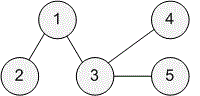Competitions

# Tree Distances I

Time limit 1 second
Memory limit 128 MiB

You are given a tree consisting of n nodes.

Your task is to determine for each node the maximum distance to another node.

## Input data

The first line contains an integer n\:(1 \le n \le 2 \cdot 10^5) — the number of nodes. The nodes are numbered 1, 2, .., n.

Then there are n − 1 lines describing the edges. Each line contains two integers a and b\:(1 \le a, b \le n) — there is an edge between nodes a and b.

## Output data

Print n integers: for each node 1, 2, .., n the maximum distance to another node.## Examples

Input example #1
5
1 2
1 3
3 4
3 5

Output example #1
2 3 2 3 3